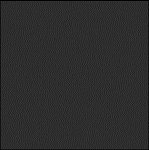# プログラミング ＋ アカデミック + 何か面白いこと

2. 0 viewPocket

# What I want to do

I’ll output PGM file with Haskell to do Image Processing.

# Program

```main = do
let pgm_type = "P5\n"
let pgm_comment = "# PGM type grayscale image\n"
let pgm_size = (255,255)
let pgm_max_brightness = 255

let pgm_size_string = (show (fst pgm_size)) ++ " " ++ (show (snd pgm_size)) ++ "\n"
let pgm_max_brightness_string = (show pgm_max_brightness) ++ "\n"
let pgm_header = pgm_type ++ pgm_comment ++ pgm_size_string ++ pgm_max_brightness_string
-- Main

Compile and run.

```\$ghc pgm.hs
\$./pgm
\$cat test.pgm
P5
# PGM type grayscale image
255 255
255
```
Pocket